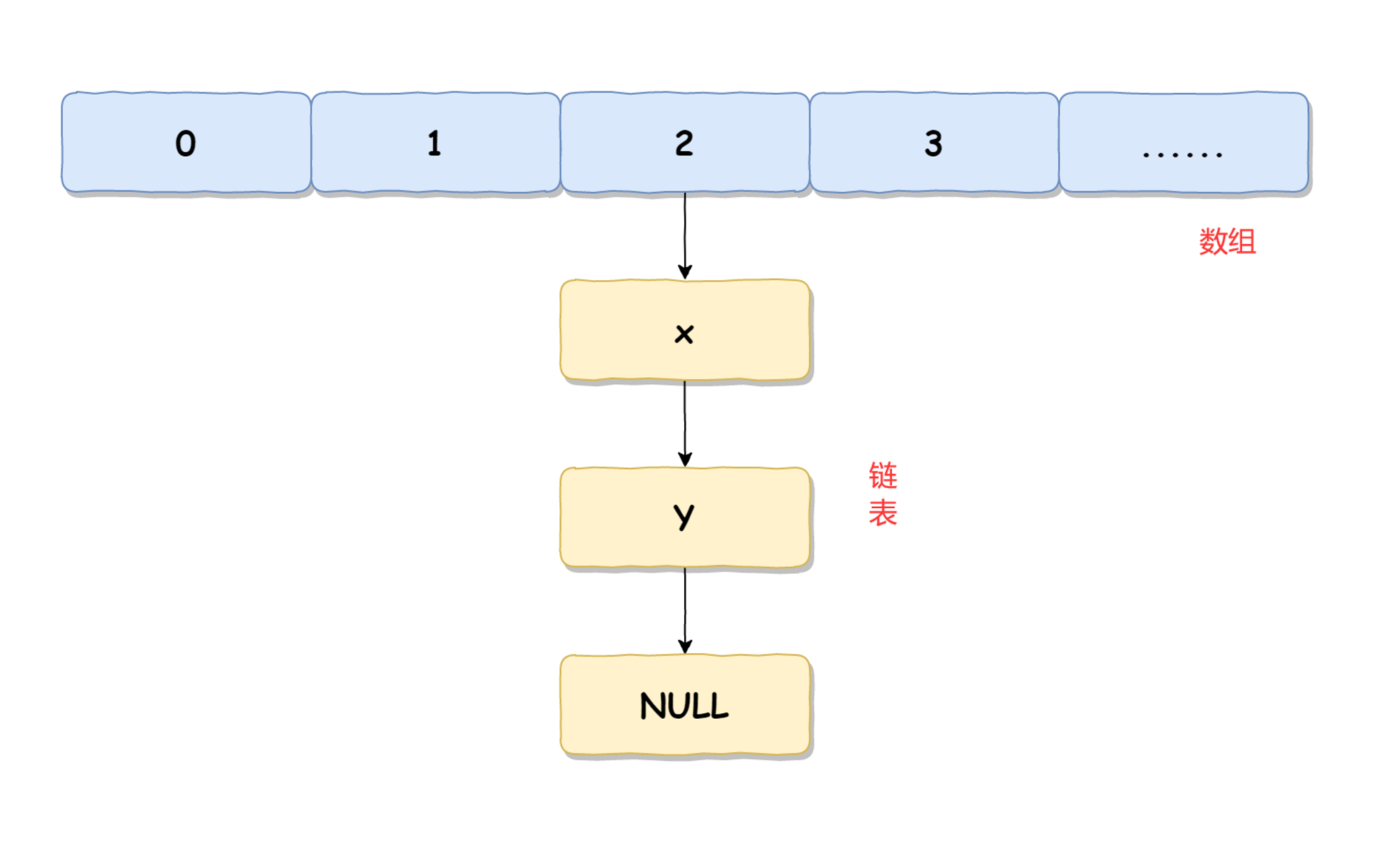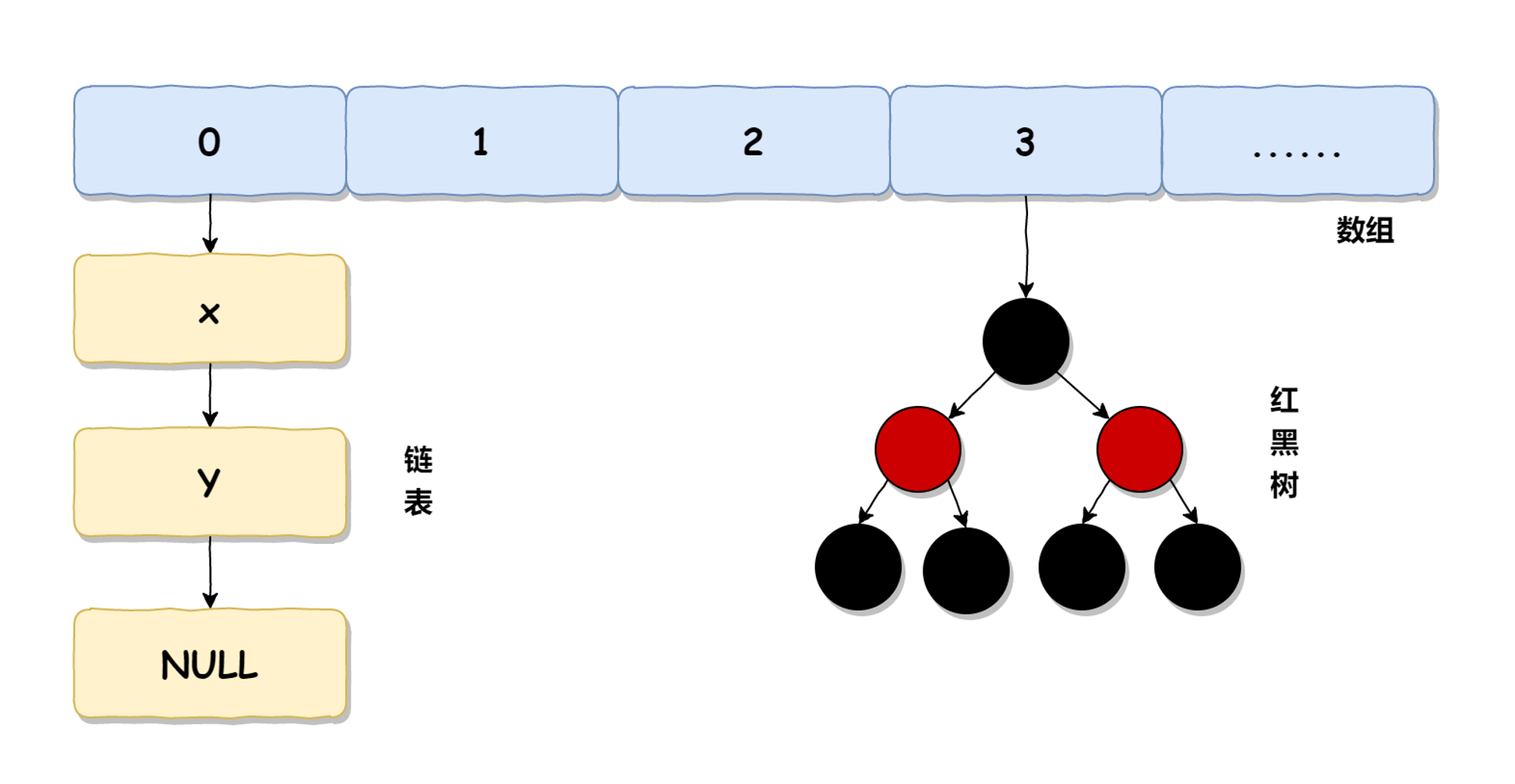墨滴2022/01/13  阅读：33  主题：萌绿

面试突击15：说一下HashMap底层实现？以及插入流程？

HashMap 是使用频率最高的数据类型之一，同时也是面试必问的问题之一，尤其是它的底层实现原理，既是常见的面试题又是理解 HashMap 的基石，所以重要程度不言而喻。

HashMap 底层实现

HashMap 在 JDK 1.7 和 JDK 1.8 的底层实现是不一样的，在 JDK 1.7 中，HashMap 使用的是数组 + 链表实现的，而 JDK 1.8 中使用的是数组 + 链表或红黑树实现的。 HashMap 在 JDK 1.7 中的实现如下图所示：HashMap 在 JDK 1.8 中的实现如下图所示：我们本文重点来学习主流版本 JDK 1.8 中的 HashMap。HashMap 中每个元素称之为一个哈希桶（bucket），哈希桶包含的内容有 4 个：

• hash 值
• key
• value
• next（下一个节点）

HashMap 插入流程

HashMap 元素新增的实现源码如下（下文源码都是基于主流版本 JDK 1.8）：

public V put(K key, V value) {
// 对 key 进行哈希操作
return putVal(hash(key), key, value, falsetrue);
}
final V putVal(int hash, K key, V value, boolean onlyIfAbsent,
boolean evict)

{
Node<K,V>[] tab; Node<K,V> p; int n, i;
// 哈希表为空则创建表
if ((tab = table) == null || (n = tab.length) == 0)
n = (tab = resize()).length;
// 根据 key 的哈希值计算出要插入的数组索引 i
if ((p = tab[i = (n - 1) & hash]) == null)
// 如果 table[i] 等于 null，则直接插入
tab[i] = newNode(hash, key, value, null);
else {
Node<K,V> e; K k;
// 如果 key 已经存在了，直接覆盖 value
if (p.hash == hash &&
((k = p.key) == key || (key != null && key.equals(k))))
e = p;
// 如果 key 不存在，判断是否为红黑树
else if (p instanceof TreeNode)
// 红黑树直接插入键值对
e = ((TreeNode<K,V>)p).putTreeVal(this, tab, hash, key, value);
else {
// 为链表结构，循环准备插入
for (int binCount = 0; ; ++binCount) {
// 下一个元素为空时
if ((e = p.next) == null) {
p.next = newNode(hash, key, value, null);
// 转换为红黑树进行处理
if (binCount >= TREEIFY_THRESHOLD - 1// -1 for 1st
treeifyBin(tab, hash);
break;
}
//  key 已经存在直接覆盖 value
if (e.hash == hash &&
((k = e.key) == key || (key != null && key.equals(k))))
break;
p = e;
}
}
if (e != null) { // existing mapping for key
V oldValue = e.value;
if (!onlyIfAbsent || oldValue == null)
e.value = value;
afterNodeAccess(e);
return oldValue;
}
}
++modCount;
// 超过最大容量，扩容
if (++size > threshold)
resize();
afterNodeInsertion(evict);
return null;
}

为什么要将链表转红黑树？

JDK 1.8 中引入了新的数据结构红黑树来实现 HashMap，主要是出于性能的考量。因为链表超过一定长度之后查询效率就会很低，它的时间复杂度是 O(n)，而红黑树的时间复杂度是 O(logn)，因此引入红黑树可以加快 HashMap 在数据量比较大情况下的查询效率。

哈希算法实现

HashMap 的哈希算法实现源码如下：

static final int hash(Object key) {
int h;
return (key == null) ? 0 : (h = key.hashCode()) ^ (h >>> 16);
}

总结

HashMap 在 JDK 1.7 时，使用的是数组 + 链表实现的，而在 JDK 1.8 时，使用的是数组 + 链表或红黑树的方式来实现的，JDK 1.8 之所以引入红黑树主要是出于性能方面的考虑。HashMap 在插入时，会判断当前链表的长度是否大于 8 且数组的长度大于 64，如果满足这两个条件就会把链表转成红黑树再进行插入，否则就是遍历链表插入。 ​

参考文档2022/01/13  阅读：33  主题：萌绿